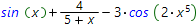# Mathematical expressions input rules## Numbers input:

One can input integer numbers in the common way, for example: 4; 18; 56
To input the negative number it is necessary to put the minus sign before the number: -19; -45; -90
Rational numbers are entered by means of the / symbol, for instance: 3/4;-5/3;5/(-19)
Decimal numbers can be entered by using the dot as the separator of integer and decimal parts of the number: 4.5;-0.4

## Variables and constants input:

Variable and constants are entered be means of latin letters, for example: x; y; z; a; b.
Constants π and e can be input as pi and e - correspondingly.
Infinity symbol is entered with two small oo latin latters or by means of the inf.
The plus infinity and the minus infinity are entered as +oo and -oo correspondingly.

Addition and subtraction are entered by means of the + and - signs correspondingly, for instance: 3+a; x+y; 5-4+t; a-b+4; ATTENTION! No spaces are needed between the operands, for example the input: x + a - wrong, right input is x+a - without the spaces.

Math expressions input rules